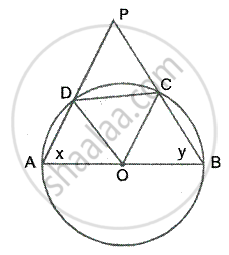Share

Abcd is a Cyclic Quadrilateral of a Circle with Centre O Such that Ab is a Diameter of this Circle and the Length of the Chord Cd is Equal to the Radius of the Circle. If Ad and Bc Produced Meet at P - ICSE Class 10 - Mathematics

Question

ABCD is a cyclic quadrilateral of a circle with centre O such that AB is a diameter of this circle and the length of the chord CD is equal to the radius of the circle. If AD and BC produced meet at P, Show that APB = 60°

SolutionIn a circle, ABCD is a cyclic quadrilateral in which
AB is the diameter and chord CD is equal to the radius of the circle

To prove - ∠APB = 60°
Construction – Join OC and OD
Proof – Since chord CD = CO = DO      [radii of the circle]
∴ ΔDOC is an equilateral triangle
∴ ∠DOC = ∠ODC = ∠DCO = 60°

Let ∠A = x and ∠B = y

Since OA =OB = OC = OD        [radii of the same circle]

∴ ∠ODA = ∠OAD = x
∠OCB = ∠OBC = y and

∴ ∠AOD = 180° - 2x and ∠BOC = 180°- 2y

But AOB is a straight line
∴ ∠AOD+∠BOC+∠COD = 180°
⇒ 180° - 2x +180° - 2y + 60° =180°
⇒ 2x +2y = 240°
⇒ x + y = 120°
But ∠A +∠B+∠P = 180°  [Angles of a triangle]
⇒ 120° + ∠P =180°
⇒ ∠P= 180° - 120°
⇒ ∠P = 60°

Hence  ∠APB= 60°

Is there an error in this question or solution?
Solution Abcd is a Cyclic Quadrilateral of a Circle with Centre O Such that Ab is a Diameter of this Circle and the Length of the Chord Cd is Equal to the Radius of the Circle. If Ad and Bc Produced Meet at P Concept: Cyclic Properties.
S